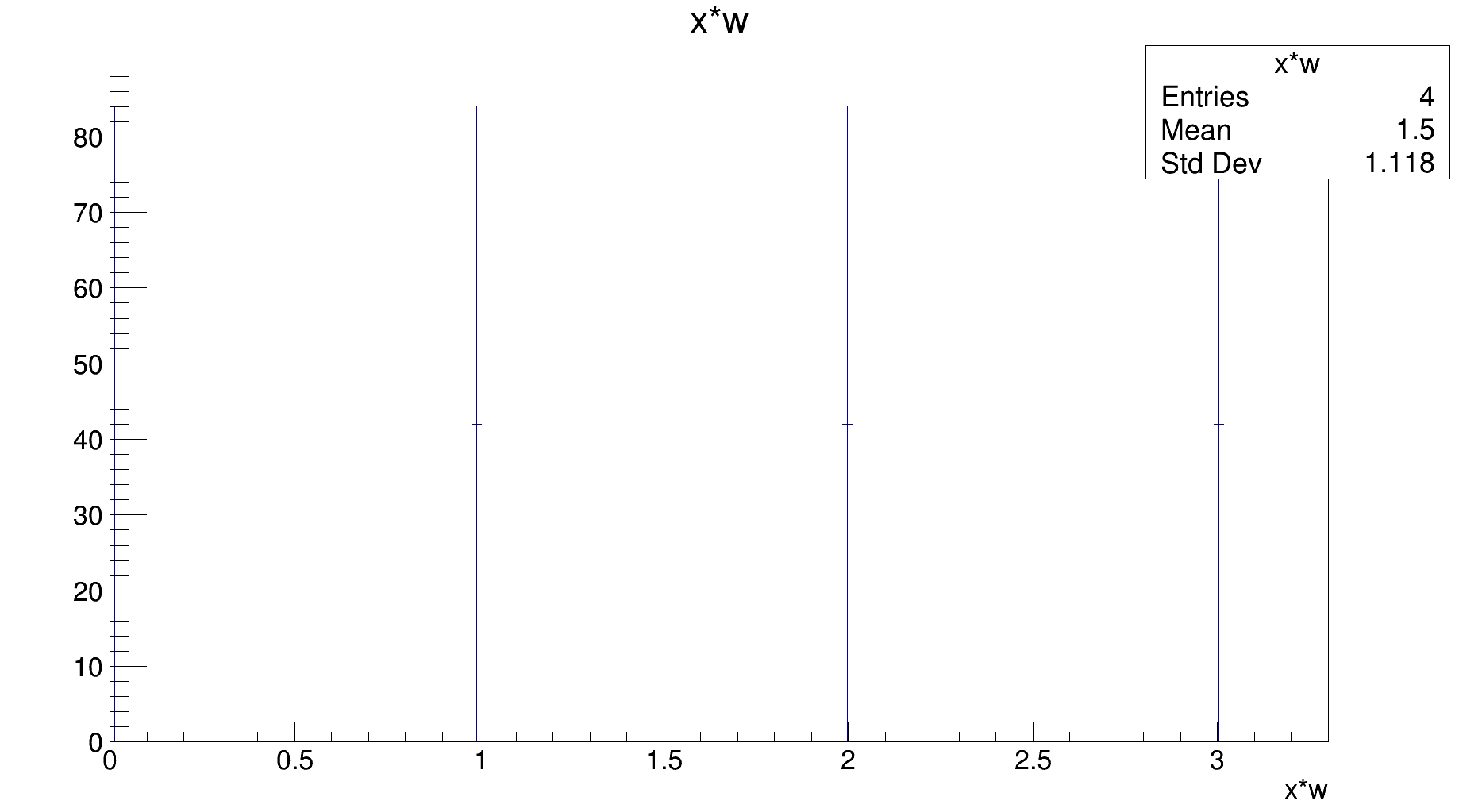# Rdataframe define column of same constant value

Hello,

I am trying to define a column where every entry is the same constant number. I tried something like this:

``````    const = some float number
ROOT.gInterpreter.Declare('float const = float(TPython::Exec("const"));')
``````

and then
d.Define(“new_column”,“return const;”)

But when I go to print this column to check the values it is just a column of float 1s. Where have I gone wrong?

Derek

ROOT Version: 6.17/01
Platform: Ubuntu 18.04 LTS
Compiler: Not Provided

Hi Derek,

``````my_number = 42
mydf_2 = mydf.Define("new_column", str(my_number))
# ... more code
``````

Cheers,
P

Pnine,

I intend to use this column as weights to a Histo1D. While your solution does display the correct information, I don’t think it can be used as weights and I am unaware of a way to then cast the string to a float in the column definition. Do you have any thoughts on this?

Derek

Hi,
what @Pnine suggests should be equivalent to `mydf.Define("new_column", "4.5")` which defines a (C++ double) column with the value you want, and that you can use to fill a `Histo1D`.

I do not understand why what you tried first did not work, however. Maybe @etejedor or @swunsch have an idea.

Cheers,
Enrico

Hi all!

So here’s a minimal reproducer:

``````import ROOT
x = 42.0
ROOT.gInterpreter.Declare('float x = float(TPython::Exec("x"));')
print(ROOT.x)
``````

This should print 42.0 but it prints 1.0. No further investigation doneLet’s have a look!

Best
Stefan

Edit:

Even nicer reproducer:

``````import ROOT
x = 42.0
ROOT.gInterpreter.ProcessLine('cout << TPython::Exec("x") << endl;')
``````

Hi again,

Ok, the problem is a missunderstanding of `TPython::Exec`, see here:

https://root.cern/doc/master/classTPython.html#a97c25640f2354724d46471235f26d180

It does not (!) return the result of the statement but a bool whether the thingy was interpreted nicely or not.

Try the following:

``````import ROOT
x = 42.0
ROOT.gInterpreter.ProcessLine('cout << TPython::Exec("print(x)") << endl;')
``````

This prints

``````42.0
1
``````

, the first 42.0 is the print of `x` and the second the bool (as integer).

Does this help?

Best
Stefan

Thanks Stefan!

I guess @Derek_Brehm should have used `TPython::Eval` instead?

I’m still a little bit confused about what the actual problem is, feel free to post a reproducer! I think you want to do something like this?

``````import ROOT
w = 42.0
df = ROOT.RDataFrame(4).Define("x", "float(rdfentry_)").Define("w", str(w))
h = df.Histo1D("x", "w")
h.Draw()
``````

This returns the (correct) plot below.Best
Stefan

Exactly! See the code below (note that you have to cast the returned object of `TPython::Eval` since it’s a `TPyReturn` object):

``````import ROOT
x = 42.0
ROOT.gInterpreter.ProcessLine('cout << float(TPython::Eval("x")) << endl;')
``````

Best
Stefan

@Derek_Brehm wants to Define a column that contains a constant, but the constant is stored in a python variable.

The original solution OP proposed was correct, except for the `TPython::Exec/Eval` mismatch.

The solution proposed by Pnine also looks correct.

@Derek_Brehm, I think we can close this as solved?

Yes! I definitely did not understand differences between TPython::Exec and TPython::Eval so thanks to all for clearing this up for me!

Derek

This topic was automatically closed 14 days after the last reply. New replies are no longer allowed.